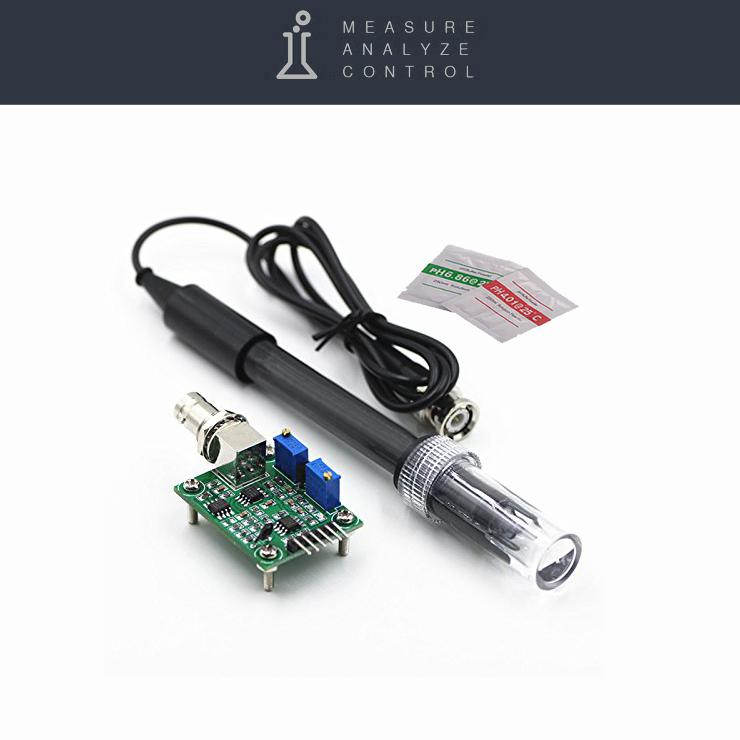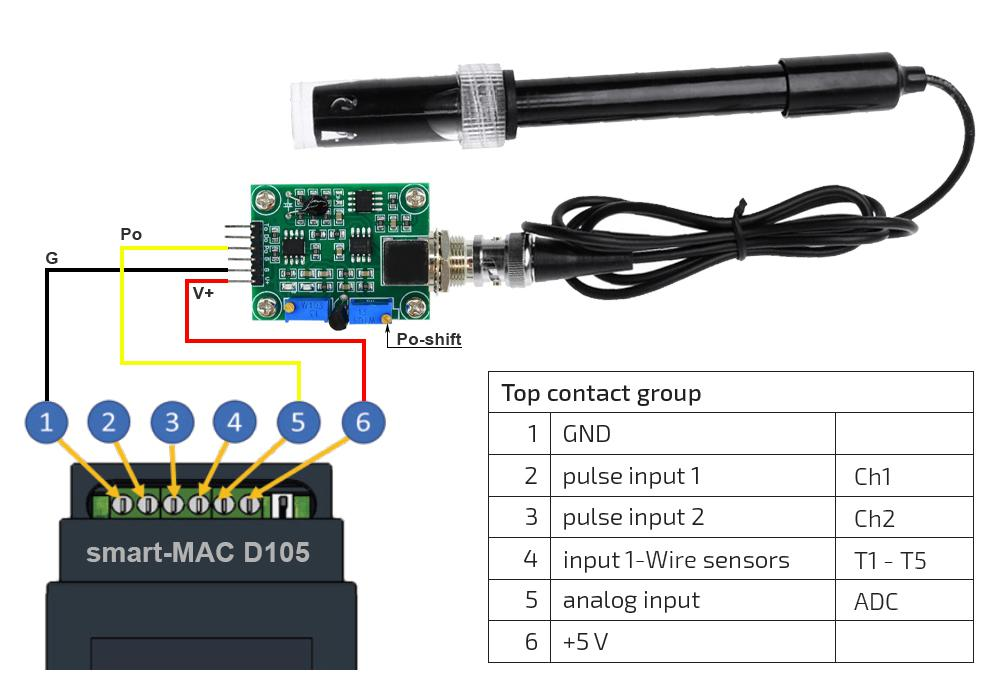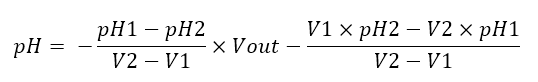# D105: Connecting the pH sensor

### PH sensorA sensor can be connected to the smart-MAC D105 universal meter to determine the pH level in aquariums, pools and water treatment systems.

The pH sensor is supplied with an interface board that converts the signal on the sensor to an analog voltage.

Wiring diagram:

• connect the black wire (G) of the sensor to terminal 1: GND (Ground or -5V)
• connect the red wire (V +) of the sensor to terminal 6: + 5V
• connect the yellow wire (Po) of the sensor to terminal 5: ADCConnection diagram pH sensor to smart-MAC D105

#### PH meter calibration

Before use and periodically it is necessary to calibrate the pH sensor. Prepare a buffer solution by dissolving calibration bags in a container of 250 ml of distilled water and let the container stand for 7-10 minutes. Measure and record the result of the pH level according to the table on the bags and the corresponding voltage on the sensor. Measure for two pH levels adjusted for solution temperature.

1. output voltage V1 at pH1 = 4.01;
2. output voltage V2 at pH2 = 6.02;

As a result, we obtain two points for constructing a linear equation for finding the pH level depending on the output voltage Vout, we recall the school course of algebra.For example, as a result of measurements, the following results were obtained:

pH1 = 4.00; V1 = 3.61 V
pH2 = 6.86; V2 = 3.17V

The linear equation for finding the pH level depending on the output voltage Vout will take the form:

```pH = 27.47 - 6.50 * Vout
```

#### Widgets settings on the Dashboard

Add a widget indicator, chart or table to the board.

In the "Arithmetic operation" section, select the function f (x) and write down the linear function obtained during the calibration process to calculate the pH depending on the voltage at the sensor.

`27.47 - 6.50 * d.ADC`#### Set up notifications on the device and control the built-in relay.

To configure notifications on the D105 device and automatically control the built-in relay by pH level, you need to determine the formula for calculating the voltage at the analog input Vout at the appropriate pH level.

`Vout = (27.47 - pH) / 6.50`

Where:

pH is the level at which an alert should come or a relay trip;
Vout - voltage at the analog input, with the corresponding pH level.